# AP Board 9th Class Maths Solutions Chapter 11 Areas Ex 11.2

AP State Syllabus AP Board 9th Class Maths Solutions Chapter 11 Areas Ex 11.2 Textbook Questions and Answers.

## AP State Syllabus 9th Class Maths Solutions 11th Lesson Areas Exercise 11.2Question 1.
The area of parallelogram ABCD is 36cm2. Calculate the height of parallelogram ABEF if AB = 4.2 cm.Solution:
Area of □ABCD = 36 cm2
AB = 4.2 cm
then □ABCD = AB X Height
[ ∵ base x height]
36 = 4.2 x h
∴ h = $$\frac { 36 }{ 4.2 }$$
But □ ABCD and □ ABEF are on the same base and between the same parallels.
∴ □ABCD = □ABEF
□ABEF = base x height = AB x height
∴ height = $$\frac { 36 }{ 4.2 }$$ = 8.571cm 5

Question 2.
ABCD is a parallelogram. AE is perpendicular on DC and CF is perpendicular on AD. If AB = 10 cm; AE = 8 cm and CF = 12 cm. Find AD.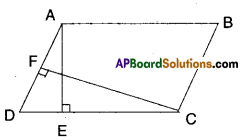Solution:
Area of parallelogram = base x height
AB x AE = AD x CF
⇒ 10 x 8 = 12 x AD
⇒ AD = $$\frac{10 \times 8}{12}$$ = 6.666 ……….Question 3.
If E, F, G and H are respectively the midpoints of the sides AB, BC, CD and AD of a parallelogram ABCD, show that ar (EFGH) = $$\frac { 1 }{ 2 }$$ ar (ABCD).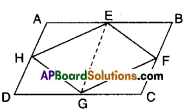Solution:
Given that □ABCD is a parallelogram.
E, F, G and H are the midpoints of the sides.
Join E, G.
Now
ΔEFG and □EBCG he on the same base EG and between the same parallels
EG // BC.
∴ ΔEFG = $$\frac { 1 }{ 2 }$$□EBCG ……………(1)
Similarly,
ΔEHG = $$\frac { 1 }{ 2 }$$□EGDA …………….(2)
ΔEFG + ΔEHG = $$\frac { 1 }{ 2 }$$ □EBCG + $$\frac { 1 }{ 2 }$$ □EGDA
□EFGH = $$\frac { 1 }{ 2 }$$[□EBCG +□ EGDA]
□EFGH = $$\frac { 1 }{ 2 }$$ [□ABCD]
Hence proved.

Question 4.
What figure do you get, if you join ΔAPM, ΔDPO, ΔOCN and ΔMNB in the example 3 ?
Solution: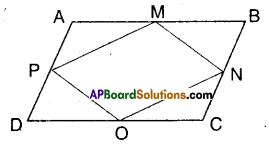□ABCD is a rhombus.
M, N, O and P are the midpoints of its sides. By joining ΔAPM, ΔDPO, ΔOCN and ΔMNB we get the figure shown by shaded region.Question 5.
P and Q are any two points lying on the sides DC and AD .respectively of a parallelogram ABCD. Show that ar (ΔAPB) = ar (ΔBQC).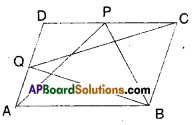Solution:
ΔAPB and □ABCD are on the same base
AB and between the same parallel lines
AB//CD.
∴ ΔAPB = $$\frac { 1 }{ 2 }$$ □ABCD …………… (1)
Also ΔBCQ and □BCDA are on the same base BC and between the same paral¬lel lines BC//AD.
∴ ΔBCQ = $$\frac { 1 }{ 2 }$$ □BCDA …………….. (2)
But □ABCD and □BCDA represent same parallelogram.
∴ΔAPB = ΔBCQ [from (1) & (2)]

Question 6.
P is a point in the interior of a parallelogram ABCD. Show that
i) ar (ΔAPB) + ar (ΔPCD) = $$\frac { 1 }{ 2 }$$ar(ABCD)
(Hint : Through P, draw a line paral¬lel to AB)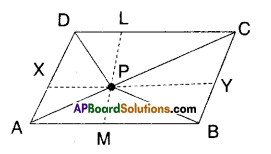Solution:
□ABCD is a parallelogram.
P is any interior point.
Draw a line $$\overline{\mathrm{XY}}$$ parallel to AB through P.
Now ΔAPB = $$\frac { 1 }{ 2 }$$ □AXYB ……………(1)
[∵ ΔAPB, □AXYB lie on the same base AB and beween AB//XY]
Also ΔPCD = $$\frac { 1 }{ 2 }$$ □CDXY ………………… (2)
[ ∵ ΔPCD; □CDXY lie on the same
base CD and between CD//XY]
Adding (1) & (2), we get
Δ APB + ΔPCD = $$\frac { 1 }{ 2 }$$ □AXYB + $$\frac { 1 }{ 2 }$$ □CDXY
= $$\frac { 1 }{ 2 }$$ [□ AXYB + □ CDXY] [from the fig.)
= $$\frac { 1 }{ 2 }$$ □ABCD
Hence Proved.(ii) ar (ΔAPD) + ar (ΔPBQ = ar (ΔAPB) + ar (ΔPCD)
Solution:
ΔAPD + ΔPBC = $$\frac { 1 }{ 2 }$$ □AMLD + $$\frac { 1 }{ 2 }$$ □BMLC
= $$\frac { 1 }{ 2 }$$ [□AMLD + $$\frac { 1 }{ 2 }$$ BMLC].
= $$\frac { 1 }{ 2 }$$ □ABCD
= ΔAPB +ΔPCD [from(i)]
Hence proved.
[ ∵ ΔAPD, □AMLD are on same base AD and between same parallels AD and LM]

Question 7.
Prove that the area of a trapezium is half the sum of the parallel sides mul¬tiplied by the distance between them.
Solution: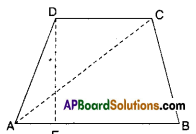Let □ABCD is a trapezium; AB//CD and
DE ⊥ AB
= $$\frac { 1 }{ 2 }$$ AB x DE + $$\frac { 1 }{ 2 }$$ DC x DE
[∵ Δ = $$\frac { 1 }{ 2 }$$ x base x height]
= $$\frac { 1 }{ 2 }$$ x DE [AB + DC]
Hence proved.Question 8.
PQRS and ABRS are parallelograms and X is any point on the side BR.
Show that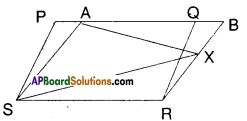i) ar (PQRS) = ar (ABRS)
Solution:
□PQRS and □ABRS are on the same base SR and between the same parallels SR//PB.
∴ □PQRS = □ABRS

ii) ar (ΔAXS) = $$\frac { 1 }{ 2 }$$ ar (PQRS)
Solution:
From (1) □PQRS = □ABRS
And □ABRS and ΔAXS are on the same base AS and between the same paral¬lels AS//BR.
∴ ΔAXS = $$\frac { 1 }{ 2 }$$ □ABRS
= $$\frac { 1 }{ 2 }$$ □PQRS from (1)
Hence proved.Question 9.
A farmer has a held in the form of a parallelogram PQRS as shown in the figure. He took the midpoint A on RS and joined it to points P and Q. In how many parts the field is divided ? What are the shapes of these parts ? The farmer wants to sow groundnuts which are equal to the sum of pulses and paddy. How should he sow ? State reasons.Solution:
From the figure ΔAPQ, □PQRS are on the same base PQ and between the same parallels PQ//SR.
∴ ΔAPQ = $$\frac { 1 }{ 2 }$$□PQRS
⇒ □PQRS – AAPQ = $$\frac { 1 }{ 2 }$$□PQRS
∴ $$\frac { 1 }{ 2 }$$□PQRS = ΔASP + ΔARQ
∴ The farmer may sow groundnuts on ΔAPQ region.
The farmer may sow pulses on ΔASP region.
The farmer may sow paddy on ΔARQ region.Question 10.
Prove that the area of a rhombus is equal to half of the product of the diagonals.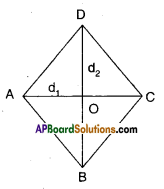Solution:
Let □ABCD be a Rhombus.
d1, d2 are its diagonals bisecting at ‘O’
We know that d1 ⊥ d1
∴ ΔABC = $$\frac{1}{2} \mathrm{~d}_{1} \cdot\left(\frac{\mathrm{d}_{2}}{2}\right)$$
[∵ base = d1; height = \frac{\mathrm{d}_{2}}{2}[/latex] ]
ΔADC = $$\frac{1}{2} \mathrm{~d}_{1} \cdot\left(\frac{\mathrm{d}_{2}}{2}\right)$$
[ ∵ base = d1;height= $$\frac{\mathrm{d}_{2}}{2}$$]
∴ □ABCD = ΔABC + ΔADC
= $$\frac{\mathrm{d}_{1} \mathrm{~d}_{2}}{4}+\frac{\mathrm{d}_{1} \mathrm{~d}_{2}}{4}=\frac{\mathrm{d}_{1} \mathrm{~d}_{2}}{2}$$
Hence Proved.